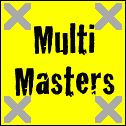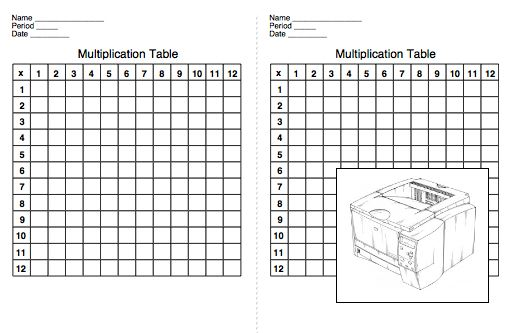Back to Mr. Anker TestsChart Champions
It is recommended that students complete and master each multiplication activity before moving on to the next.

 Multiplication Chart 12's, 5's & 10's Multiplication Chart 23's, 4's & 11's Multiplication Chart 36's, 7's & 8's Multiplication Chart 47's, 8's & 9's
 Multiplication Chart 59's, 10's, 11's & 12's Multiplication Chart 6A20 Chart Squares (Timed) Multiplication Chart 6B20 Chart Squares (Timed) Multiplication Chart 6C20 Chart Squares (Timed)
 Multiplication Chart 6D20 Chart Squares (Timed) Multiplication Chart 6E20 Chart Squares (Timed) Multiplication Chart 6FThe Full Chart (Timed)-Answers Remain- Multiplication Chart 6GThe Full Chart (Timed)-Answers Disappear-

More Multiplication Tests
 Multiplication2's Multiplication3's Multiplication4's Multiplication5's
 Multiplication6's Multiplication7's Multiplication8's Multiplication9's
 Multiplication10's Multiplication11's Multiplication12's MissingDecimal Factors
 MultiplicationDoubles Multiplication6, 7, 8, & 9's MultiplicationRandom 3 RandonFactors
 MultiplicationSet A MultiplicationSet B MultiplicationSet C MultiplicationSet D
 MultiplicationSet E Mental MultiplyingSet 1 Mental MultiplyingSet 2 Mental MultiplyingSet 3